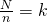# Sampling & Survey #6 – Systematic SamplingFor today, it is going to be a short and simple session on Systematic Sampling, We can effectively consider systematic sampling as a proxy for simple random sampling.

We start by taking(rounding up, if necessary), then draw a random number R from 1 to k. Then proceed to draw a systematic sample in periodic cycle.

Firstly, its not SRS as not every possible group of units have the same probability of being the sample.

Secondly, it is a form of cluster sampling since we divide our population in k clusters and draw one cluster. More on it in part 10.

Thirdly, some populations might be in some order, for example, alphabetical, increasing or decreasing order. If the population is in random order, the systematic sample will behave much like an SRS>

Lastly, systematic sample may be more representative, may have smaller variance than SRS. But this is highly dependent on what samples we are looking at, for instance if we are studying accounts receivable, then a systematic sample may have more variability (contains some large and some small amounts) than SRS (contains all large or small amounts). Having a periodic or cyclical order, will result in systematic sampling giving a less representative sample.

Sampling & Survey #1 – Introduction
Sampling & Survey #2 – Simple Probability Samples
Sampling & Survey #3 – Simple Random Sampling
Sampling & Survey #4 – Qualities of estimator in SRS
Sampling & Survey #5 – Sampling weight, Confidence Interval and sample size in SRS
Sampling & Survey #6 – Systematic Sampling
Sampling & Survey #7 – Stratified Sampling
Sampling & Survey # 8 – Ratio Estimation
Sampling & Survey # 9 – Regression Estimation
Sampling & Survey #10 – Cluster Sampling
Sampling & Survey #11 – Two – Stage Cluster Sampling
Sampling & Survey #12 – Sampling with unequal probabilities (Part 1)
Sampling & Survey #13 – Sampling with unequal probabilities (Part 2)
Sampling & Survey #14 – Nonresponse

Not readable? Change text.